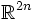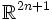# Hard Whitney embedding theorem

This article is about an embedding theorem, viz about sufficient conditions for a given manifold (with some additional structure) to be realized as an embedded submanifold of a standard space (real or complex projective or affine space)
View a complete list of embedding theorems

## Statement

A compact connected differential manifold of dimension$n$ can be embedded inside$\R^{2n}$.

This is an improvement on the Whitney embedding theorem, which states that any compact connected differential manifold of dimension$n$ can be embedded inside$\R^{2n+1}$ and immersed inside$\R^{2n}$.